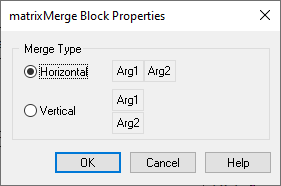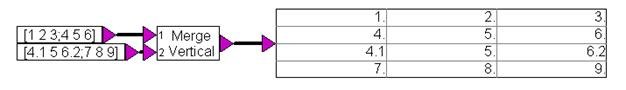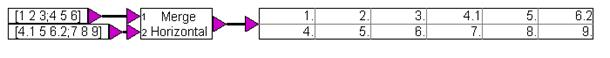## matrixMerge

Block Category: Matrix Operation

Description: The matrixMerge block merges two matrices into one. For a horizontal merge, the number of rows in each input matrix must be the same; for a vertical merge, the number of columns in each input matrix must be the same.Horizontal: Represents the arguments horizontally.

Vertical: Represents the arguments vertically.

#### Example

Two identical matrices are input into two matrixMerge blocks, where the upper block merges the matrices vertically and the lower block merges them horizontally.